$$\def\RR{\bf R} \def\real{\mathbb{R}} \def\bold#1{\bf #1} \def\d{\mbox{Cord}} \def\hd{\widehat \mbox{Cord}} \DeclareMathOperator{\cov}{cov} \DeclareMathOperator{\var}{var} \DeclareMathOperator{\cor}{cor} \newcommand{\ac}{\left\{#1\right\}}$$

## Big Complex Data, Causality, and Brain Pathways

### Xi (Rossi) Luo

Brown University
Department of Biostatistics
Center for Statistical Sciences
Computation in Brain and Mind
Brown Institute for Brain Science
ABCD Research Group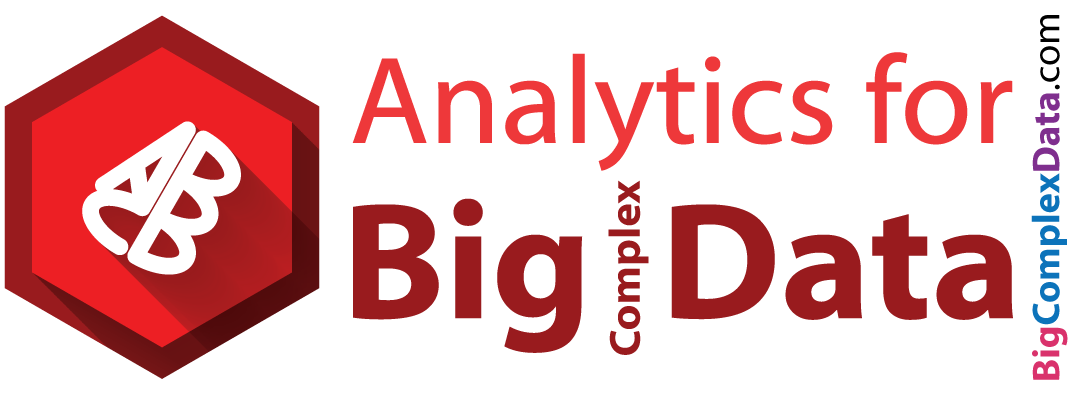The Big Data and Medical Imaging Workshop
Sanya, CHINA

December 27, 2016

Funding: NIH R01EB022911; NSF/DMS (BD2K) 1557467; NIH P20GM103645, P01AA019072, P30AI042853; AHA

## CoauthorYi Zhao
(4rd Yr PhD Student, on job market this year)
Receipient of multiple student awards, including the 2015 ENAR distinguished paper

Brown University

## Math Problem: Infer Causality from Obs. Data Only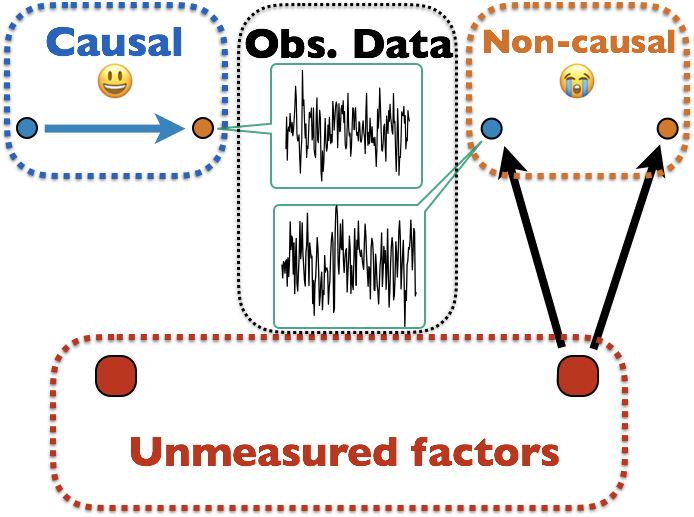Many unmodeled factors: vascular, respriatory, mind wandering, ...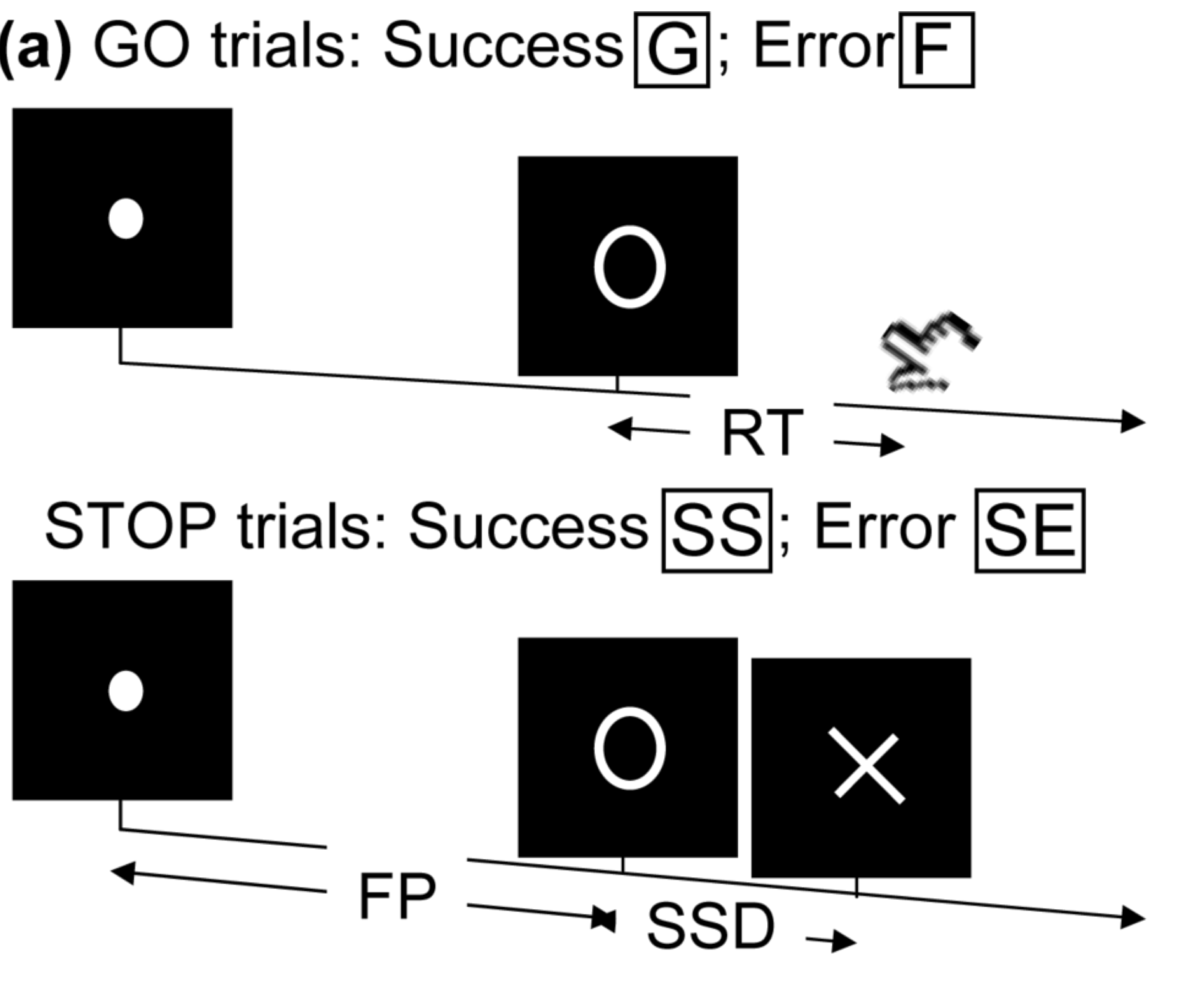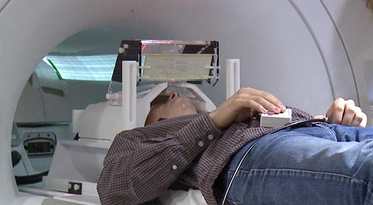• press button if "go";
• withhold pressing if "stop"
• Not resting-state: "rest" in scanner

Goal: causal mechanisms from stimuli to motor cortex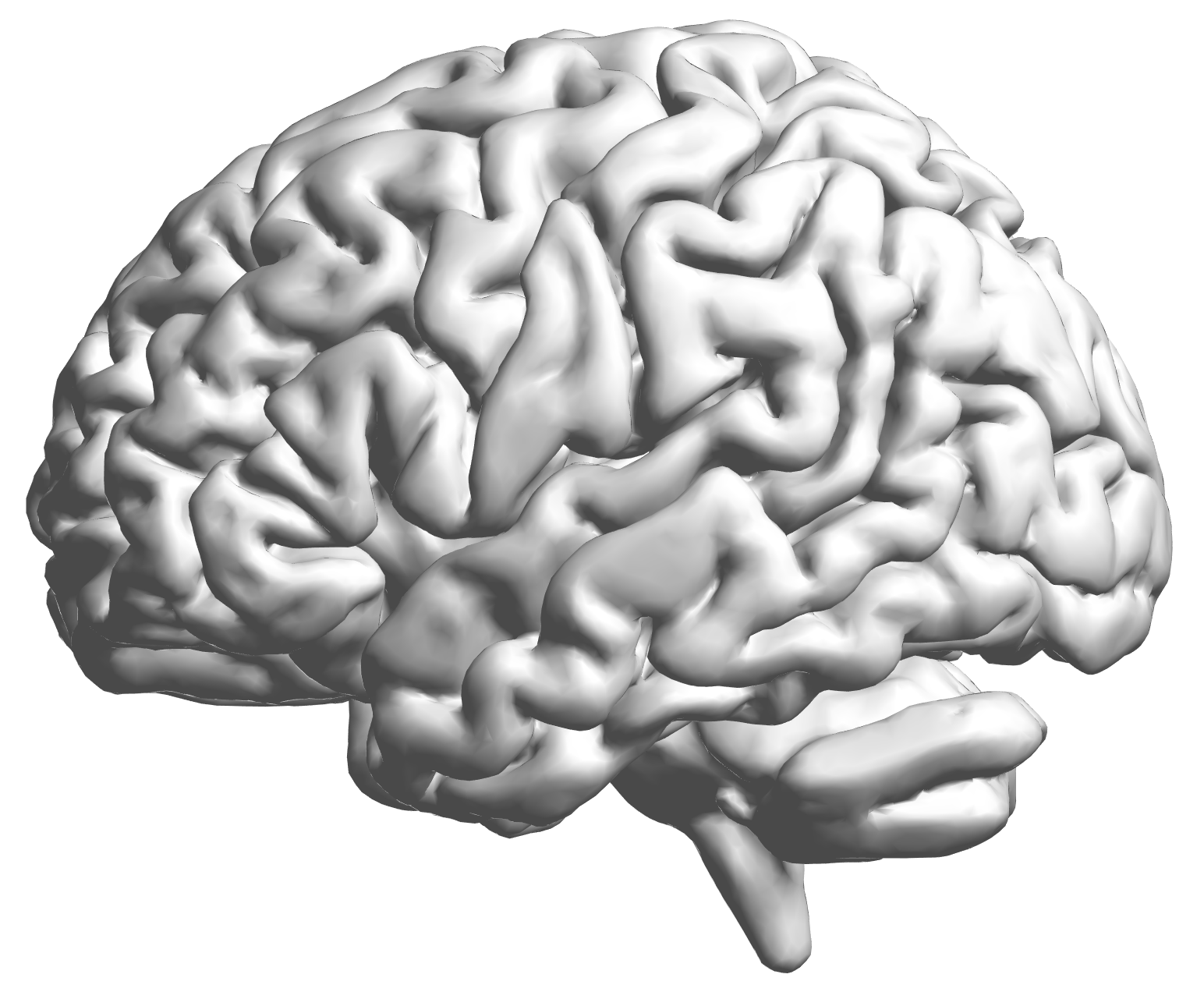fMRI data: blood-oxygen-level dependent (BOLD) signals from each cube/voxel (~millimeters), $10^5$ ~ $10^6$ voxels in total.

## Raw Data: Motor Region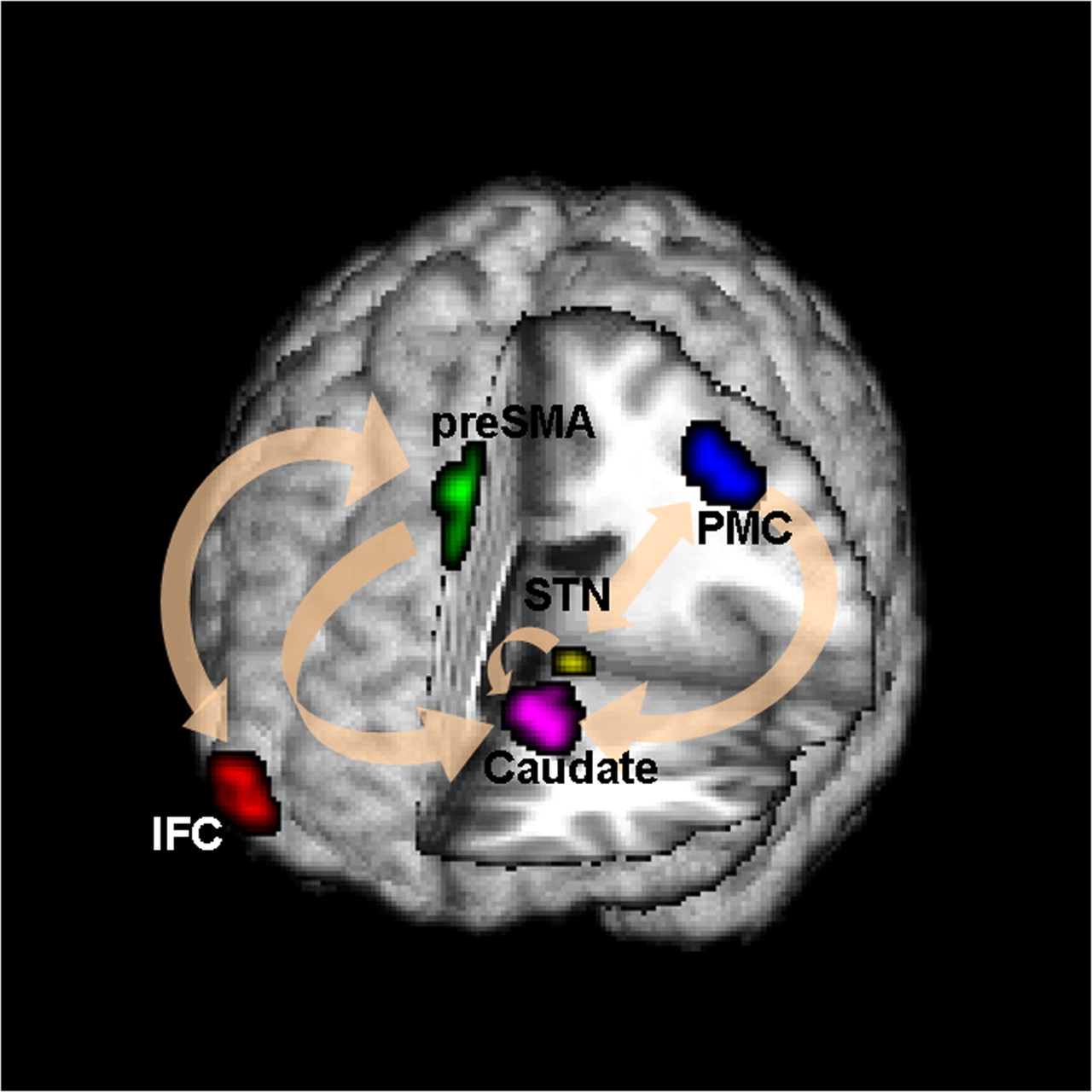Time (seconds)

Black: fMRI BOLD activity
Blue: stop onset times; Red: go onset times

## fMRI Studies

Sub 1, Sess 1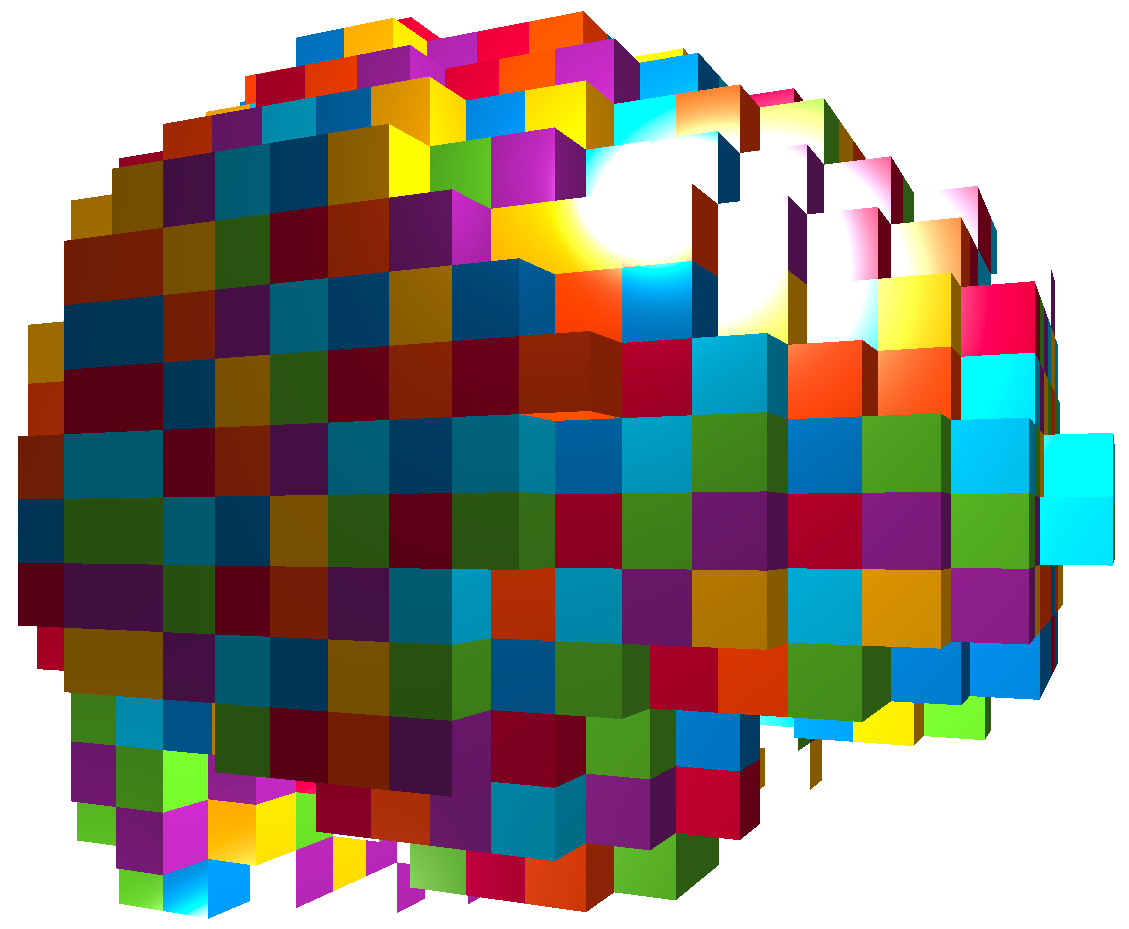Time 12~300

Sub i, Sess jSub ~100, Sess ~4Our data: 98 subjects × 4 sessions × 100 trials × 2 regions

Question: can "big and complex" fMRI data be helpful?

## Brain Networks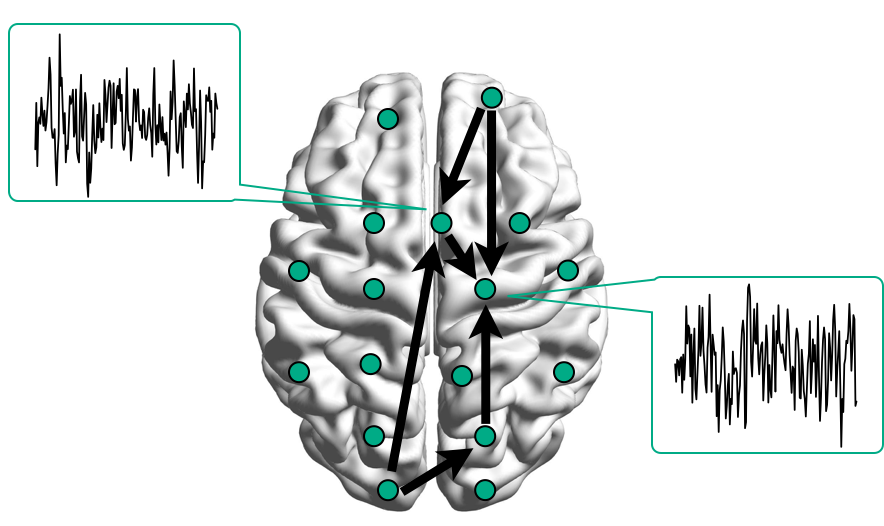Functional/Effective Connectivity

nodes/connections removed to enhance visualization

## Network Model with Stimulus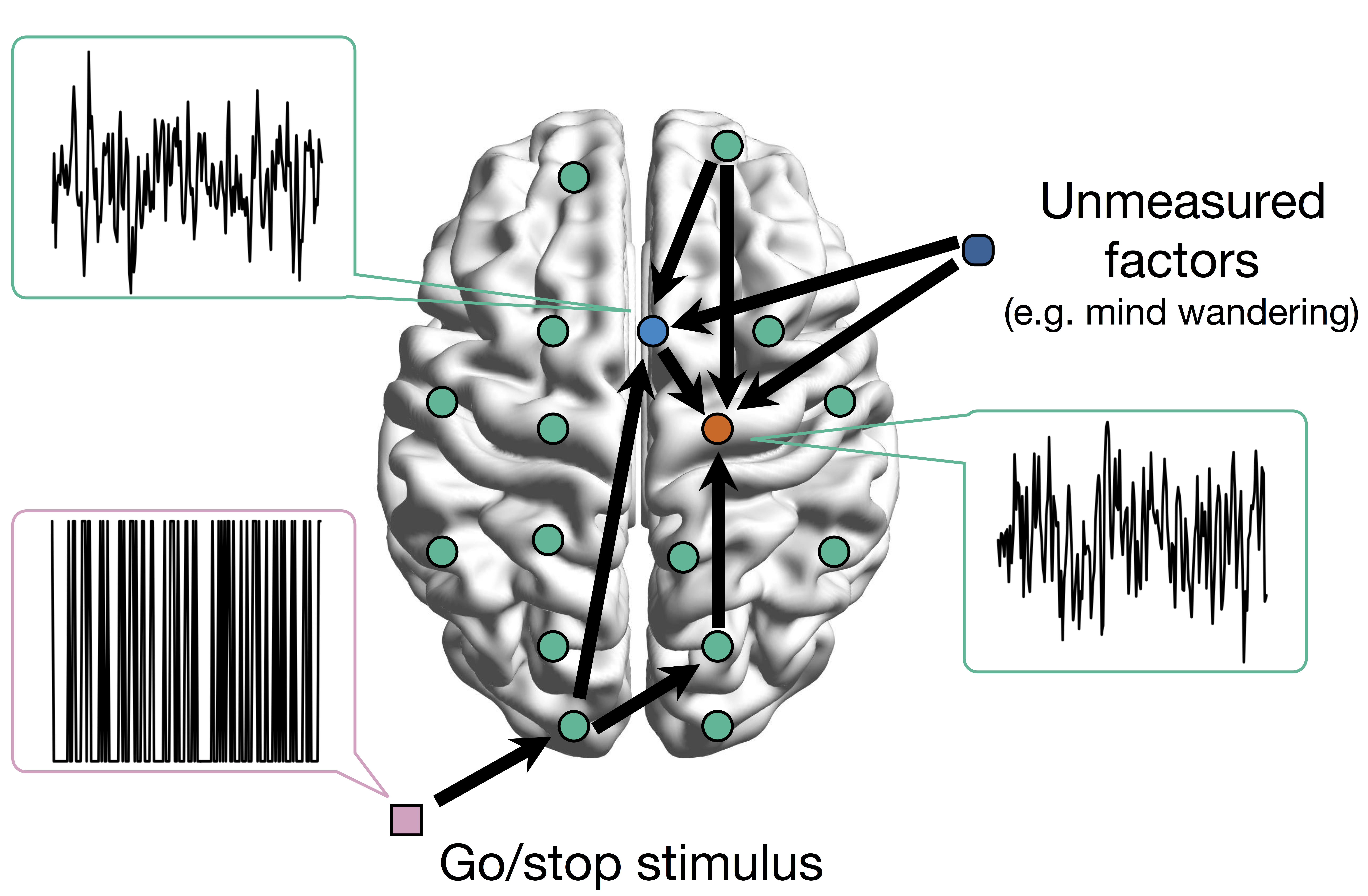Goal: quantify effects stimulipreSMAPMC regions Duann, Ide, Luo, Li (2009). J of Neurosci

# Model

## Mediation Analysis and SEM\begin{align*}M &= Z a + \overbrace{U + \epsilon_1}^{E_1}\\ R &= Z c + M b + \underbrace{U g + \epsilon_2}_{E_2}, \quad \epsilon_1 \bot \epsilon_2\end{align*}
• Indirect effect: $a \times b$; Direct effect: $c$
• Correlated errors: $\delta = \cor(E_1, E_2) \ne 0$ if $U\ne 0$

## Mediation Analysis in fMRI

• Mediation analysis (usually assuming $U=0$)
• Baron&Kenny, 86; Sobel, 82; Holland 88; Preacher&Hayes 08; Imai et al, 10; VanderWeele, 15;...
• Parametric Wager et al, 09 and functional Lindquist, 12 mediation, under (approx.) independent errors
• Stimulus $\rightarrow$ brain $\rightarrow$ user reported ratings, one brain mediator
• Assuming $U=0$ between ratings and brain
• Multiple mediator and multiple pathways
• Dimension reduction by arXiv1511.09354Chen, Crainiceanu, Ogburn, Caffo, Wager, Lindquist, 15
• Pathway Lasso penalization Zhao, Luo, 16
• This talk: causal estimation under $U\ne 0$ (its effect size $\delta \ne 0$) when modeling two brain regions## Existing Approaches for $\delta \ne 0$

• Assuming $\delta=0$
• Assumption "too strong" for most cases Imai et al, 10
• Sensitivity plot: "guessing" $\delta$
• Simplify models: e.g. $c=0$ via instrumental variable
• Adjust (e.g. motion) if possible Sobel, Lindquist, 14
• Use Bayesian prior or regularizationPositive or negative effects depending on subjective choice of $\delta$

# Method

## Our Approach: Step 1

• Bivariate single-level model \begin{align*}\begin{pmatrix}M & R\end{pmatrix} & = \begin{pmatrix}Z & M\end{pmatrix} \begin{pmatrix}a & c\\ 0 & b \end{pmatrix} + \begin{pmatrix}E_{1} & E_{2}\end{pmatrix}\\ (E_1, E_2) & \sim MVN(\boldsymbol{0}, \boldsymbol{\Sigma}) \quad \boldsymbol{\Sigma}=\begin{pmatrix}\sigma_{1}^{2} & \delta\sigma_{1}\sigma_{2}\\ \delta\sigma_{1}\sigma_{2} & \sigma_{2}^{2} \end{pmatrix} \end{align*}
• Estimate $(a,b,c,\boldsymbol{\Sigma})$ via ML (a lot of handwaving)
• We solve an optimization with constraints
• Different than running two regressions

## Causal Interpretation

• Prove causal using potential outcomes Neyman, 23; Rubin, 74
• Causal inference intuition $$Z \rightarrow \begin{pmatrix} M \\ R \end{pmatrix}$$
• Others assume (e.g. randomized brain stimulation) $$r_{i}\left(z_{i}^{\prime},m_{i}\right)\bot m_{i}\left(z_{i}\right)|Z_{i}=z_{i}$$
• We do not need this assumption given $\delta$

## Theory

• Theorem: Given $\delta$, unique maximizer of likelihood, expressed in closed form
• Theorem: Given $\delta$, our estimator is root-n consistent and efficient
• Bias (and variance) depends on $\delta$

[Stigler 2007]

# "Tragedy" of ML

Theorem: The maximum profile likelihood value is the same for every $\delta \in (-1,+1)$.• Likelihood provides zero info about $\delta$
• Cannot simply apply priors on $\delta$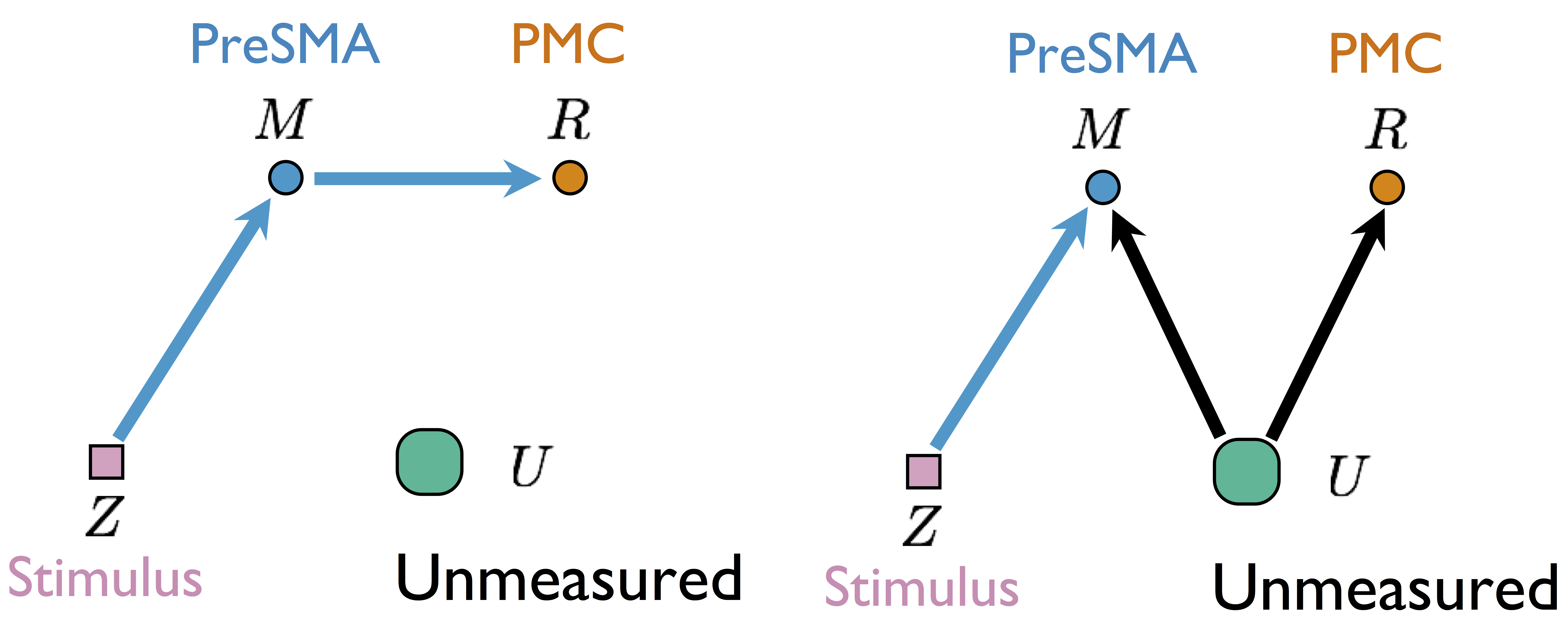Two different models generate same single-trial BOLD activations if only observing $Z$, $M$, and $R$

without measuring $U$

## Our Approach: Step 2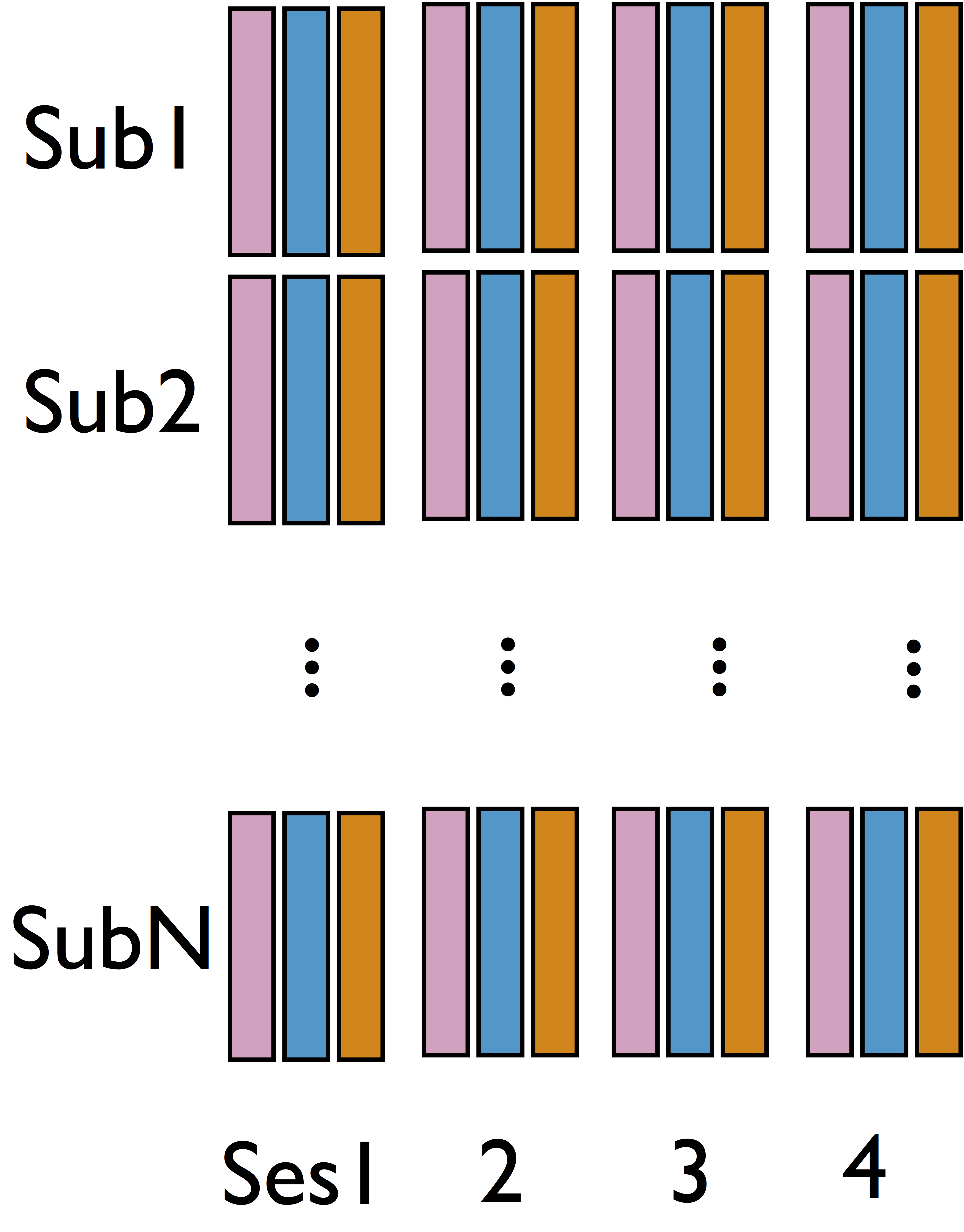• Cannot identify $\delta$ from single sub and single sess (see our theorem)
• Intuition: leverage complex data structure to infer $\delta$

## Step 2: Some Details

• Step 1 model for each sub and each sess $$\begin{pmatrix}{M}_{ik} & {R}_{ik}\end{pmatrix}=\begin{pmatrix}{Z}_{ik} & {M}_{ik}\end{pmatrix}\begin{pmatrix}{a}_{ik} & {b}_{ik}\\ 0 & {c}_{ik} \end{pmatrix}+\begin{pmatrix}{E}_{1_{ik}} & {E}_{2_{ik}}\end{pmatrix}$$
• Limited variability in $\delta$ across sub/sess
• Random effect model cf AFNI, FSL, SPM, and etc $$\begin{pmatrix}{A}_{ik}\\ {B}_{ik}\\ {C}_{ik} \end{pmatrix}=\begin{pmatrix}{A}\\ {B}\\ {C} \end{pmatrix}+\begin{pmatrix}\alpha_{i}\\ \beta_{i}\\ \gamma_{i} \end{pmatrix}+\begin{pmatrix}\epsilon_{ik}^{{A}}\\ \epsilon_{ik}^{{B}}\\ \epsilon_{ik}^{{C}} \end{pmatrix}=b+u_{i}+\eta_{ik}$$

## Option 2: Integrated Modeling

• Optimize all parameters in joint likelihood \begin{align*} &\sum_{i=1}^{N}\sum_{k=1}^{K}\log\Pr\left(R_{ik},M_{ik}|Z_{ik},\delta,b_{ik},\sigma_{1_{ik}},\sigma_{2_{ik}}\right)\quad \mbox{Data}\\ & + \sum_{i=1}^{N}\sum_{k=1}^{K}\log\Pr\left(b_{ik}|u_{i},b,\boldsymbol{\Lambda}\right)\quad \mbox{Subject variation}\\ & +\sum_{i=1}^{N}\log\Pr\left(u_{i}|\boldsymbol{{\Psi}}\right) \quad \mbox{Prior}\end{align*}
• Large computation: $5NK + 3N + 11 > 2000$ paras

## Algorithm

Theorem: The joint likelihood is conditional convex for groups of parameters.
Algorithm: block coordinate descent with projections.

Leverage conditional convexity to reduce computation

details in our paper

## Theory for SEM and Confounding

Theorem: Asymptotically, the joint multilevel likelihood has a unique maximum and the maximizer is consistent for $\delta$.

Contributions: data-driven estimation of confounding and consistency in SEM

# Simulations

## Method Comparison

• Single level models
• BKBaron, Kenny, 86: assuming $\delta = 0$
• CMA-$\delta$ (our single-level method): assuming $\delta = 0$
• Mixed effects multilevel models
• KKBKenny et al, 03: assuming all $\delta = 0$
• CMA-ts (ours): estimating $\delta$, two-stage fitting
• CMA-h, CMA-m (ours): estimating $\delta$, large-scale fitting
• Simulate data with varying $\delta$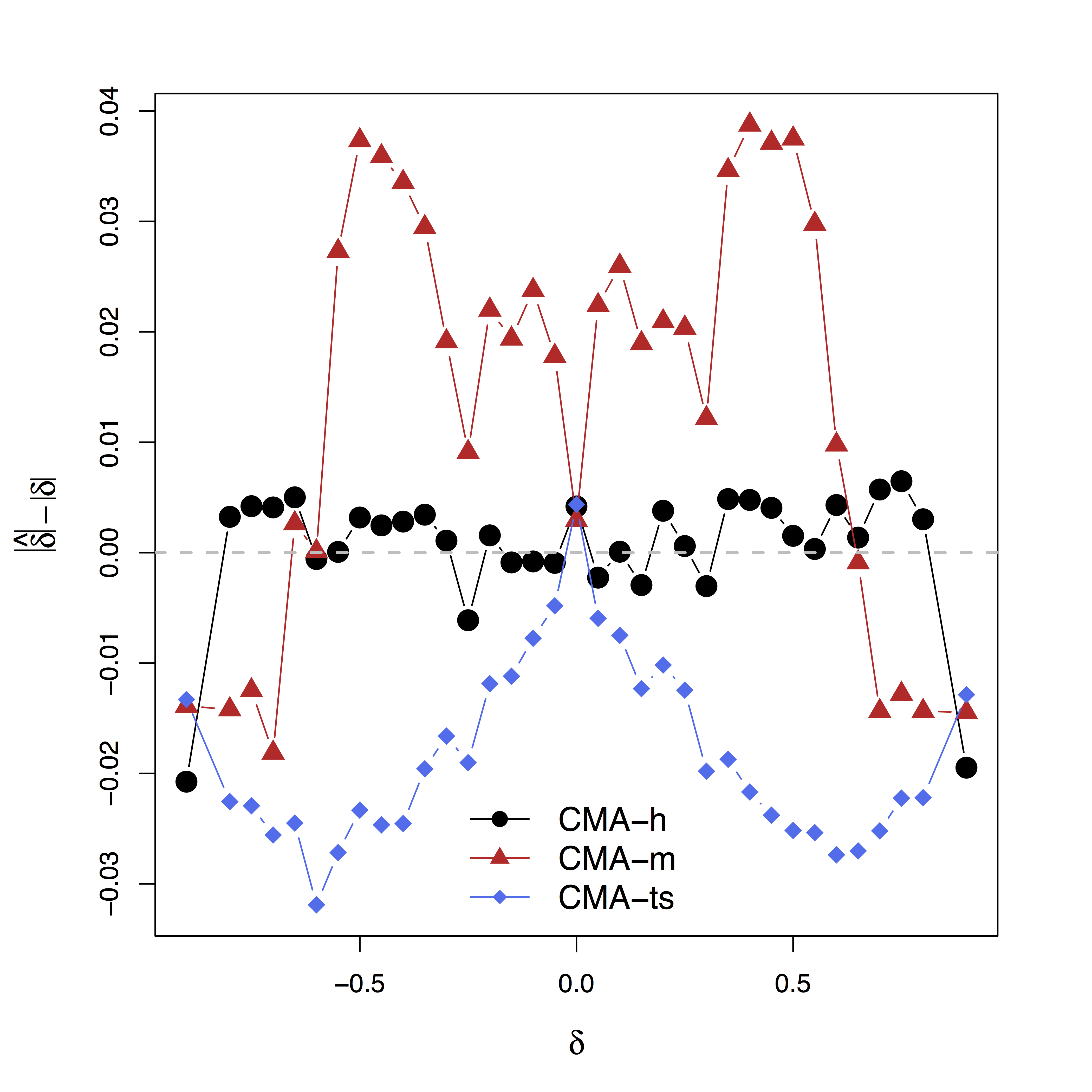Low bias for $\delta$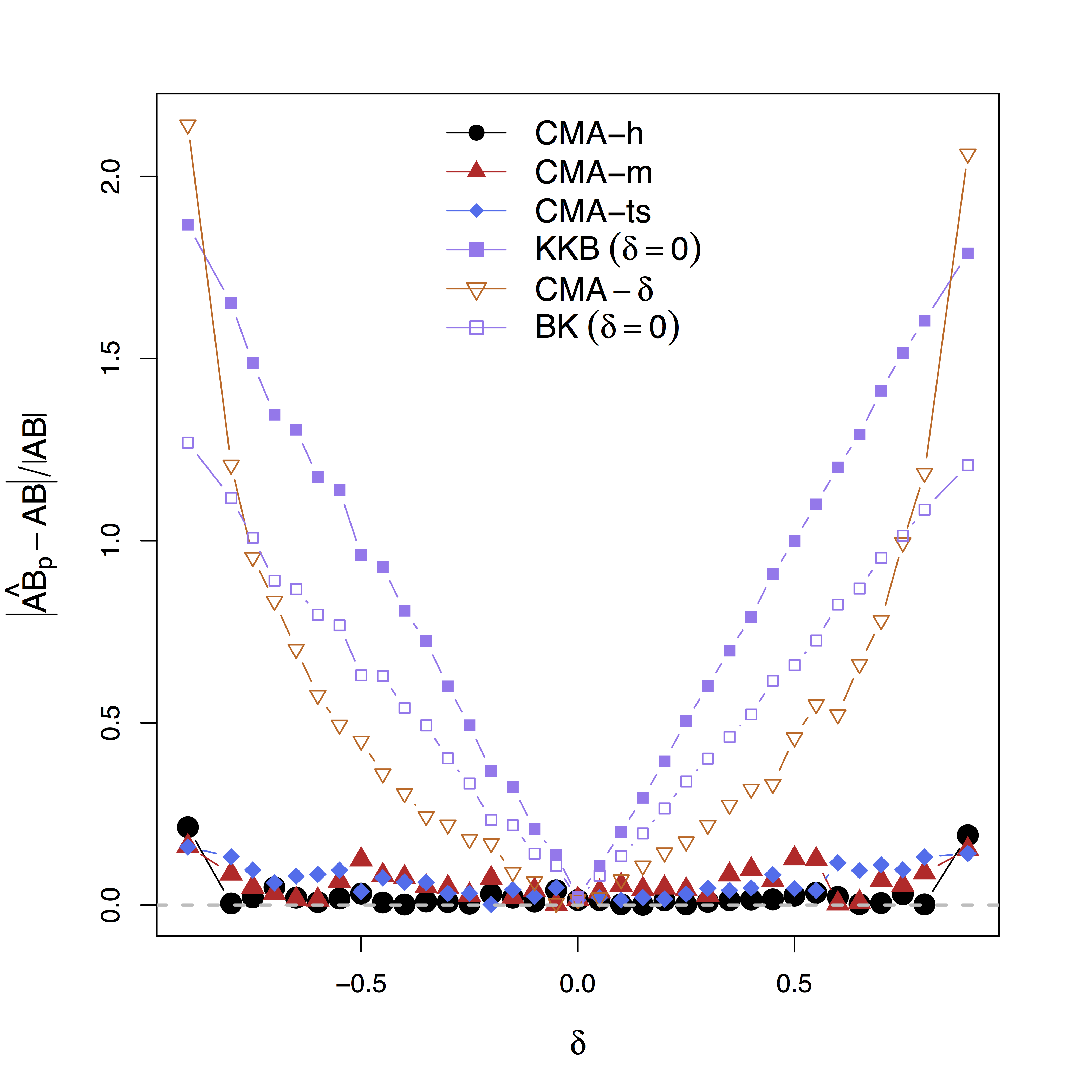Low bias for effects

Our large-scale multilevel model (CMA) performs best;
Bias can be large (100%) without estimating $\delta$

# Real Data

## Data

• Random stimuli and scalar activations for each trial
• Stimuli: go = press; stop = not pressing
• stop is expected to supress motor area (PMC)
• 98 subjects × 4 sessions × 100 trials × 2 regions
• Temporal dependence removed using whitened data and single-trial beta deconvolutionWager et al, 08

## Identifiability and Bias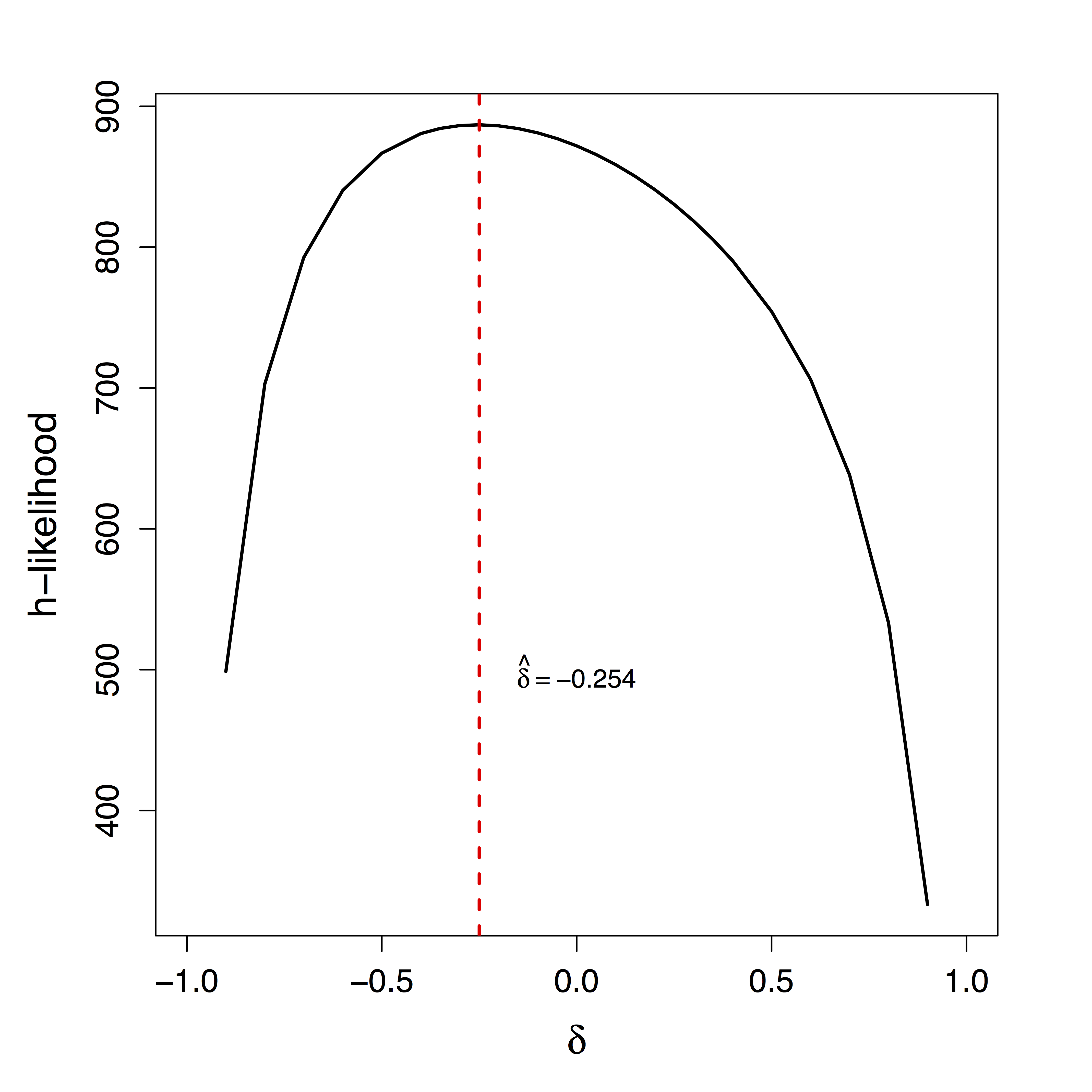Unique $\delta$ for ML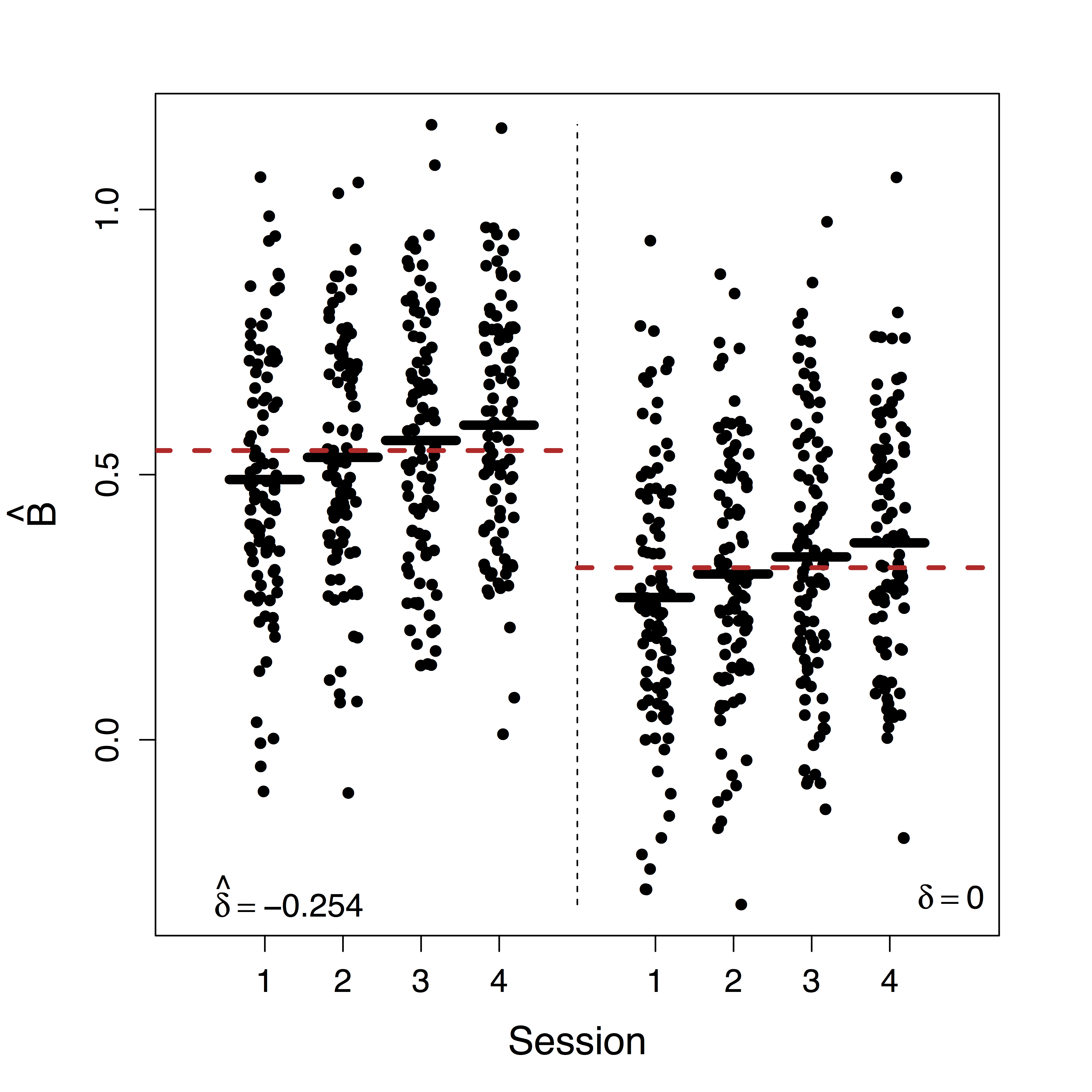Estimates doubled• Ours show stop directly supresses PMC significantly while other methods fail to recover this
• Motion (part of $U$) correction decreases our $\delta$ estimate but do not change our causal estimates

## Summary

• Leverage complex data for causal fMRI modeling
• Approach:
• large-scale causal SEM
• big multilevel data
• machine learning/optimization
• Theory: identifiability, convergence, and computation
• Result: reduced bias and causal interpretation

## Discussion

• Extensions: temporal models, functional models, multiple pathwaysPathway Lasso, our arXiv 1603.07749
• Manuscript: 2015 ENAR Student Paper Award, revision for JASA, (arXiv 1410.7217)
• R package: macc# Thank you!

## Slides at: bit.ly/sanya16